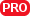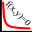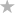#### File Exchange > DataAnalysis >    Equation SolverAuthor:
OriginLab Technical Support
11/9/2017
Last Update:
7/16/2020
1555
Total Ratings:
0
File Size:
65 KB
Average Rating:File Name:
EquationSolver.opx
File Version:
1.1
Minimum Versions:
Free
Summary:

Solve a system of nonlinear equations with varying parameters. Examine how the parameters will affect the solutions.

Screen Shot and Video:
Description:

INSTALLATION:
Download the file EquationsSolver.opx, and then drag-and-drop onto the Origin workspace. An icon will appear in the Apps Gallery window.

OPERATION:
Click the icon to open the dialog. Define the equations, set the parameter columns, enter initial guess values for independent variables. Once the equations are solved, the resulting values are stored in the same row of each set of parameters.

Dialog:

• Number of Equations:  set the number of nonlinear equations.
• Equations: Define the equations for the solver.
• Independent Variables: Once equations are entered, the app will list the varibles in the equations. Check the boxes of independent variables.
• Independent Variables Ranges: Select one column for each variables.
• Initial Values For Dependent Variables: specify initial values for each dependent varibles. User may need to change the values to obtain correct results.

Note:

NAG function nag_zero_nonlin_eqns_easy is used to solve the set of equations. More details of the function defination can be found in:

http://www.originlab.com/pdfs/nagcl25/manual/pdf/c05/c05qbc.pdf